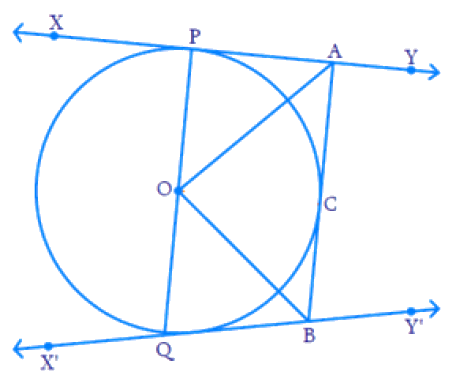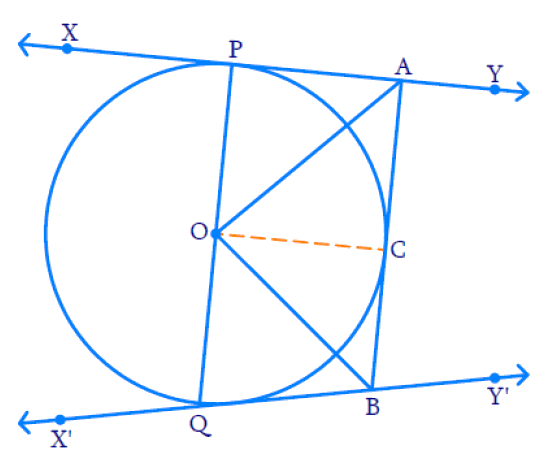# Ex.10.2 Q9 Circles Solution - NCERT Maths Class 10

Go back to  'Ex.10.2'

## Question

In Figure, $$XY$$ and $$X'Y'$$ are two parallel tangents to a circle with center $$O$$ and another tangent $$AB$$ with point of contact $$C$$ intersecting $$XY$$ at $$A$$ and $$X'Y'$$ at $$B.$$

Prove that $$\angle {AOB} = {90^ \circ }$$.Video Solution
Circles
Ex 10.2 | Question 9

## Text Solution

What is known?

•  '$$O$$' is the centre of the circle.
• $$XY$$ and $$X'Y'$$' are the two parallel tangents to the circle.
• $$AB$$ is another tangent with point of contact $$C$$, intersecting $$XY$$ at $$A$$ and $$X'Y'$$ at $$B.$$

To prove:

$$\angle {AOB} = {90^ \circ }$$

Reasoning :

Join point $$O$$ to $$C.$$In $$\Delta OPA$$ and $$\Delta OCA$$

$$OP = OC$$ (Radii of the circle are equal)

$$AP = AC$$ (The lengths of tangents drawn from an external point $$A$$ to a circle are equal.)

$$AO = AO$$  (Common)

By $$SSS$$ congruency, $$\Delta {OPA} \cong \Delta {OCA}$$

SSS Congruence Rule: If three sides of one triangle are equal to the three sides of another triangle, then the two triangles are congruent.

Steps:

Therefore, $$\angle {OPA} = \angle {OCA}$$

Similarly, $$\Delta {OCB} \cong \Delta {OBQ}$$

Therefore, $$\angle {COB} = \angle {BOQ}$$

$$PQ$$ is a diameter, hence a straight line and $$\angle {POQ} = {180^ \circ}$$

But \begin{align} \angle {P O Q} =\begin{bmatrix} \angle {P O A} + \angle {A O C}+ \\ \angle {C O B} + \angle {B O Q}\end{bmatrix}\end{align}

Therefore,
\begin{align} & \left[ \begin{array} \angle POA+\angle AOC+ \\ \angle COB+\angle BOQ \\ \end{array} \right]={{180}^{{}^\circ }} \\ & 2\angle AOC+ 2\angle COB ={{180}^{{}^\circ }}\end{align}

Therefore,
\begin{align}& \left[ \begin{array}& \angle POA=\angle AOC \;\text{and} \\\angle COB=\angle BOQ \\ \end{array} \right] \end{align}

Therefore,
$\angle AOC+\angle COB={{90}^{{}^\circ }}$

From the figure, $$\angle {A O C} + \angle {C O B} = \angle {A O B}$$

$$\therefore \quad \angle {A O B} = 90 ^ { \circ }$$

Hence Proved

Learn from the best math teachers and top your exams

• Live one on one classroom and doubt clearing
• Practice worksheets in and after class for conceptual clarity
• Personalized curriculum to keep up with school